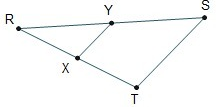# Consider △RST and △RYX. If the triangles are similar, which must be true?

Consider △RST and △RYX.
If the triangles are similar, which must be true?
A. RY/YS=RX/XT=XY/TS
B.RY/RS=RX/RT=XY/TS
C.RY/RS=RX/RT=RS/RY
D.RY/RX=RS/RT=XY/TS